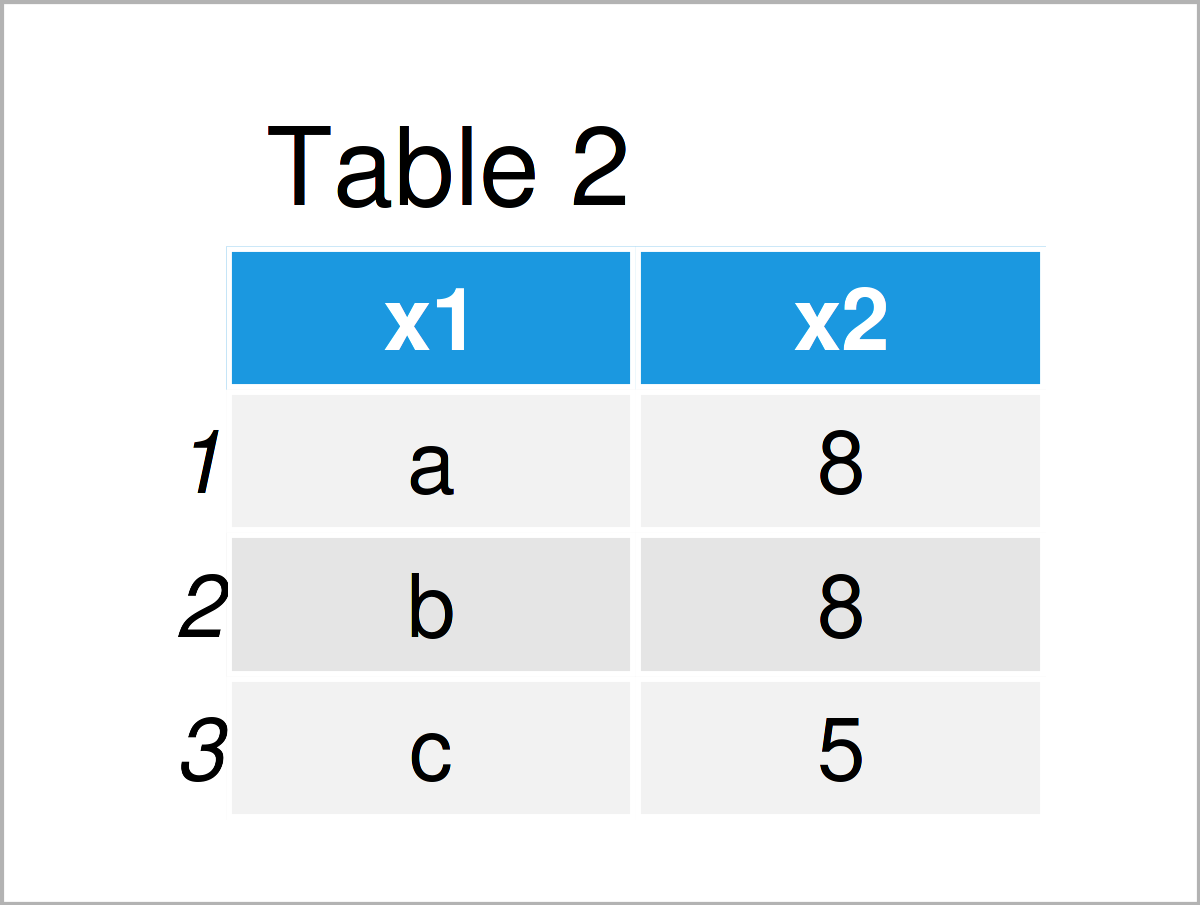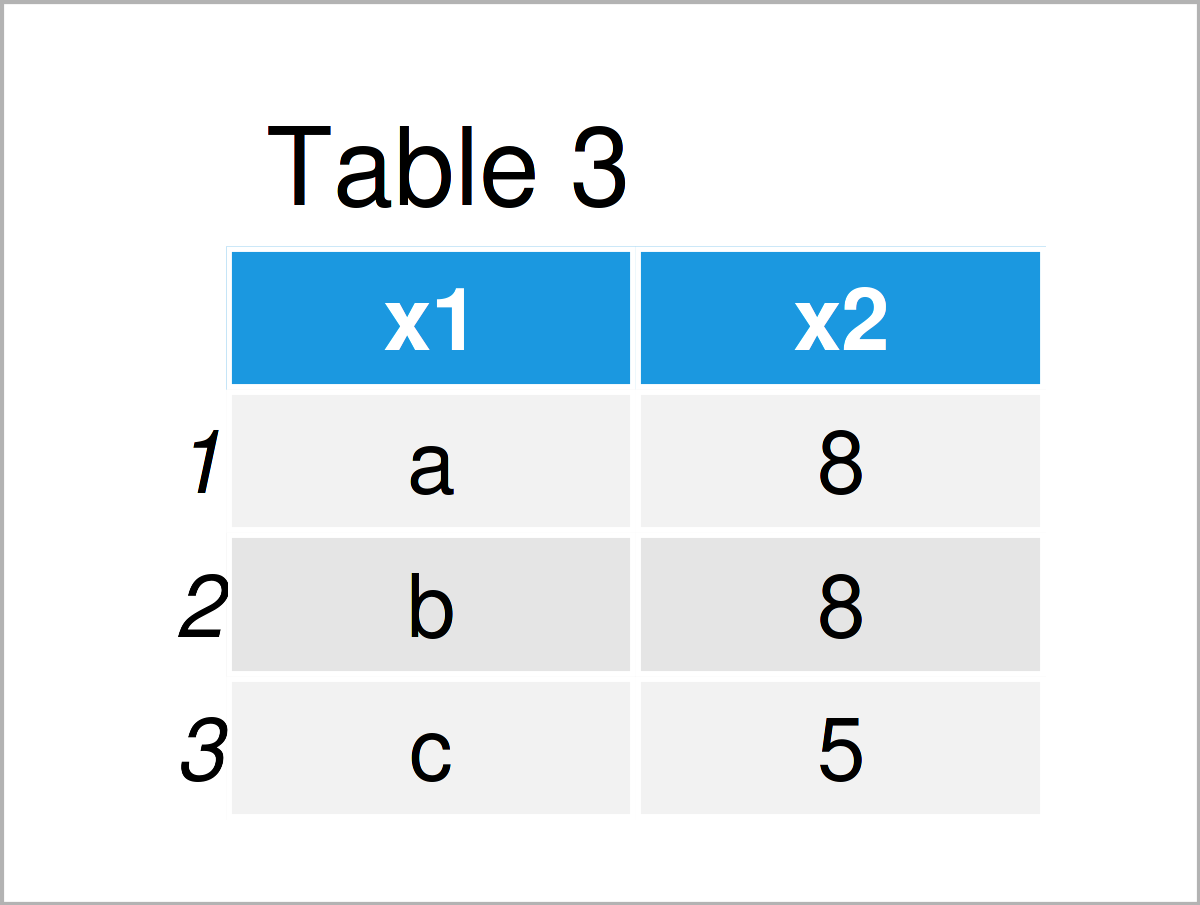# Sum Duplicate Rows in R (2 Examples)

In this article you’ll learn how to consolidate duplicate rows in R programming.

The tutorial will contain the following:

If you want to know more about these content blocks, keep reading…

## Example Data

The following data will be used as basement for this R programming tutorial:

```data <- data.frame(x1 = c("a", "b", "a", "a", "c", "b"),  # Create example data
x2 = 1:6)
data                                                      # Print example data```Table 1 shows that our exemplifying data has six rows and the two variables “x1” and “x2”. The variable x1 is a character and the variable x2 has the integer class.

## Example 1: Consolidate Duplicate Rows Using aggregate() Function

In this example, I’ll illustrate how to merge rows that are duplicated in the column x1.

For this, we can use the aggregate function that is provided by the basic installation of the R programming language as shown below:

```data_sum1 <- aggregate(x2 ~ x1, data, sum)                # Consolidate duplicates
data_sum1                                                 # Print consolidated data```In Table 2 you can see that we have created a new data set containing only three rows. All duplicates in the variable x1 have been summed up in the variable x2.

## Example 2: Consolidate Duplicate Rows Using group_by() & summarise() Functions of dplyr Package

The following code shows how to use the dplyr package to sum up duplicates.

To be able to use the functions of the dplyr package, we first have to install and load dplyr:

```install.packages("dplyr")                                 # Install & load dplyr
library("dplyr")```

Next, we can use the group_by and summarise functions to merge all duplicates in the variable x1.

Note that we are also using the as.data.frame function to create a data frame output. If you prefer to work with tibbles, you may remove this line of code.

```data_sum2 <- data %>%                                     # Consolidate duplicates
group_by(x1) %>%
dplyr::summarise(x2 = sum(x2)) %>%
as.data.frame()
data_sum2                                                 # Print consolidated data```The output of the previous R programming code is shown in Table 3 – A data frame containing exactly the same values as in Example 1.

## Video & Further Resources

Would you like to learn more about the consolidation of duplicate rows in a data frame? Then you may want to watch the following video on my YouTube channel. In the video, I’m explaining the content of this post:

In addition to the video, you could read the related tutorials on my website. You can find a selection of tutorials below:

To summarize: This tutorial has explained how to sum repeated rows in a data frame in the R programming language. Tell me about it in the comments, in case you have further questions.

Subscribe to the Statistics Globe Newsletter